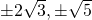# 10.8 Construct a Quadratic Equation from its Roots

It is possible to construct an equation from its roots, and the process is surprisingly simple. Consider the following:

Example 10.8.1

Construct a quadratic equation whose roots are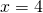and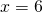.

This means that(or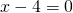) and(or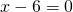).

The quadratic equation these roots come from would have as its factored form: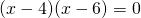All that needs to be done is to multiply these two terms together: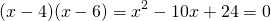This means that the original equation will be equivalent to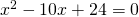.

This strategy works for even more complicated equations, such as:

Example 10.8.2

Construct a polynomial equation whose roots are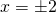and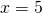.

This means that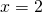(or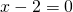),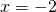(or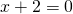) and(or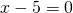).

These solutions come from the factored polynomial that looks like: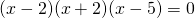Multiplying these terms together yields: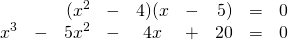The original equation will be equivalent to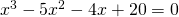.

Caveat:  the exact form of the original equation cannot be recreated; only the equivalent. For example,is the same as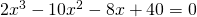,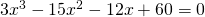,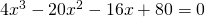,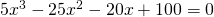, and so on. There simply is not enough information given to recreate the exact original—only an equation that is equivalent.

# Questions

Construct a quadratic equation from its solution(s).

1. 2, 5
2. 3, 6
3. 20, 2
4. 13, 1
5. 4, 4
6. 0, 9
7.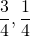8.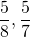9.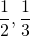10.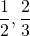11. ± 5
12. ± 1
13.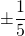14.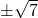15.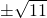16.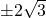17. 3, 5, 8
18. −4, 0, 4
19. −9, −6, −2
20. ± 1, 5
21. ± 2, ± 5
22.